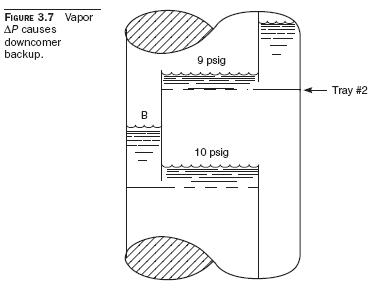# Tray Vapor-Flow Pressure Drop

We have yet to discuss the most important factor in determining the height of liquid in the downcomer. This is the pressure drop of the vapor flowing through the tray deck. Typically, 50 percent of the level in the downcomer is due to the flow of vapor through the trays.

When vapor flows through a tray deck, the vapor velocity increases as the vapor flows through the small openings provided by the valve caps, or sieve holes. The energy to increase the vapor velocity comes from the pressure of the flowing vapor. A common example of this is the pressure drop we measure across an orifice plate. If we have a pipeline velocity of 2 ft/s and an orifice plate hole velocity of 40 ft/s, then the energy needed to accelerate the vapor as it flows through the orifice plate comes from the pressure drop of the vapor itself.

Let us assume that vapor flowing through a tray deck undergoes a pressure drop of 1 psig (lb/in2 gauge). Figure 3.7 shows that the pressure below tray deck 2 is 10 psig and the pressure above tray deck 2 is 9 psig. How can the liquid in downcomer B flow from an area of low pressure (9 psig) to an area of high pressure (10 psig)? The answer is gravity, or liquid head pressure.

The height of water needed to exert a liquid head pressure of 1 psig is equal to 28 in of water. If we were working with gasoline, which has a specific gravity of 0.70, then the height of gasoline needed to exert a liquid head pressure of 1 psig would be 28 in/0.70 = 40 in of clear liquid.